xBending stiffnessEncyclopedia
The bending stiffness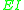of a beam
Beam (structure)
A beam is a horizontal structural element that is capable of withstanding load primarily by resisting bending. The bending force induced into the material of the beam as a result of the external loads, own weight, span and external reactions to these loads is called a bending moment.- Overview...

(or a plate
Plate theory
In continuum mechanics, plate theories are mathematical descriptions of the mechanics of flat plates that draws on the theory of beams. Plates are defined as plane structural elements with a small thickness compared to the planar dimensions . The typical thickness to width ratio of a plate...

) relates the applied bending moment
Bending Moment
A bending moment exists in a structural element when a moment is applied to the element so that the element bends. Moments and torques are measured as a force multiplied by a distance so they have as unit newton-metres , or pound-foot or foot-pound...

to the resulting deflection of the beam. It is the product of the elastic modulus
Elastic modulus
An elastic modulus, or modulus of elasticity, is the mathematical description of an object or substance's tendency to be deformed elastically when a force is applied to it...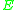of the beam material and the area moment of inertia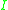of the beam cross-section. According to elementary beam theory, the relationship between the applied bending moment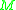and the resulting curvature
Curvature
In mathematics, curvature refers to any of a number of loosely related concepts in different areas of geometry. Intuitively, curvature is the amount by which a geometric object deviates from being flat, or straight in the case of a line, but this is defined in different ways depending on the context...of the beam is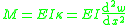whereis the deflection of the beam andthe spatial coordinate. In the literature sometimes the above definition is given with a minus sign depending on convention.

Bending Stiffness in beams is also known as Flexural Rigidity